江波
 介观物理
• # 介观物理

• ## ​自由电子气

• ### ​费米速度 $v_{F}$

• #### ​ $v_{F} = \frac{ℏ}{ m^{*} } k_{F}$

金属一般1~2*10^6(m/s)

半导体一般1~10*10^5(m/s)

• ### ​​费米能量 $E _{F}$

1. 自由电子气电子间没有相互作用，也没有势能。所以费米面是一个球面。如果存在晶格势能，费米球会发生形变。
• #### $E _{F} = \frac{ ℏ^{2} }{2m ^{*} } k_{F}^{2}$

GaAs半导体费米能2.27*10^-21(J)

• ### ​态密度 $N（ ε ）$

1. ​单位能量内态的个数。

• ### ​费米波长（德布罗意波长） $λ _{F}$

• #### ​ $λ _{F} = \frac{2 π }{ k_{F} }$

费米波长只与载流子浓度有关,与有效质量无关

• ## ​介观输运重要量

• ### ​电导率 $σ$

1. 电导率与材料形状和大小无关。

• ### ​平均自由程 $l_{e}$

1. 电子在两次大角度散射之间的平均运动距离。
2. 当材料尺寸小于平均自由程时发生弹道输运。

根据平均自由程 与系统的尺度 L 的相对大小， 介观系统分成扩散区（diffusive regime）和弹道（ballistic regime）。

• ### ​扩散系数D

1. 载流子浓度梯度导致扩散运动

• ### ​相位相干长度 $ξ _{N}$

1. 电子在退相干之前运动的距离。
2. 相干电子态并不一定是弹道输运。因为弹性散射不会导致退相干，只有非弹性散射（电声散射，电子与电子散射等）会导致退相干。后者随温度下降而减少，从而使相干长度增长。
3. 电子与电子散射虽然会引起退相干，但是不会产生电阻。因为电子的总动量并不因此而改变。
• #### ​弹道区 $L \ll l_{e}$

1. 在弹道区， 电子几乎不受杂质的散射。在极低温度下， 系统的输运性质是由费米面附近的电子所决定的。

• ## ​超导

• ### ​伦敦穿透深度 $λ _{L}$

• #### $λ _{L} =( \frac{m^{*} c^{2} }{4 π n_{s} e^{2} } ) ^{ \frac{1}{2} } ​ ​ ​ ​ ​$ ; $n_{s} 是库珀对密度$

1. 超导电子密度是相对于正常电子而言的。超导态下大部分电子都是超导电子。

• ### ​超导相干长度 $ξ _{0}$

1. 超导相干长度等于库珀对的尺寸。
• #### ​干净极限(Clean limit) $l_{e} \gg ξ _{0} ​ ​ ​ ​ ​$

1. 干净极限下，电子呈弹道输运。

• #### ​脏极限(Dirty limit) $l_{e} \ll ξ _{0} ​ ​ ​ ​ ​$

1. 在脏极限下，电子呈扩散运动。
2. 在脏极限下，有更高的临界温度和更短的相干长度。

• ### ​准粒子

• #### ​能谱 $ϵ \left( p \right)$

1. ​在不存在在磁场并且能隙在空间为常数的情况下解BdG方程得出能谱。

• #### ​弛豫时间

​从微扰（准粒子注入）消失之后，恢复到平衡态的时间。

两种模型：Schmid and Schon

• #### ​弛豫长度

在弛豫时间内扩散的长度

• ### ​Andreev 反射

• #### ​ $ϵ >Δ$

1. 能隙外的情况，可以由正常态入射，也可以从超导态入射。

• #### 例子：从正常态入射一个电子

1. 一共有四种入射情况；镜面反射b都为零。
2. 从正常态入射一个电子，沿近似原路径反射一个空穴。并透射一个电子。
3. 从正常态入射一个空穴，沿近似原路径反射一个电子。并透射一个空穴。
4. 从超导态入射一个电子，沿近似原路径反射一个空穴。并透射一个电子。
5. 从超导态入射一个空穴，沿近似原路径反射一个电子。并透射一个空穴。
6. 电子群速度与波矢k方向相同，空穴群速度与波矢k相反。

• #### ​ $ϵ <Δ$

1. 超导能隙内没有准粒子态，因此只可能由正常态入射电子或空穴。

• #### ​例子：从正常态入射一个电子

1. 一共两种情况。（没有绝缘层就没有正常反射。）
2. 从正常态入射一个电子，沿近似原路径反射一个空穴。并拽一个电子形成库珀对进入超导。
3. 从正常态入射一个空穴，沿近似原路径反射一个电子。并拽一个空穴从超导中湮灭一个库珀对。

• #### ​短结$d \ll ξ ； ℏω _{x} \gg Δ$> $ϵ$ ；

1. 能隙内只有两个驻波态：k>0时l=-1；k<0时l=0。

• #### ​ $I= I_{c}sin( ϕ ) \newline I_{c}= \frac{1}{2e R_{sh} } \frac{16ℏv_{F} }{d} e^{-d/ ξ _{N} } \newline 正常态相干长度 ξ _{N} ​$

超导的近邻效应：超导诱导正常态中的电子形成库珀对类型的关联。这个关联以ξN的特征长度衰减。正常态相干长度ξN与温度成反比。所以升温可以减小临近效应。

• ### BTK理论：​存在绝缘层 $U(x)=I δ (x) ​$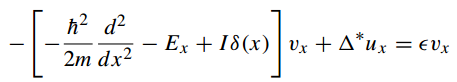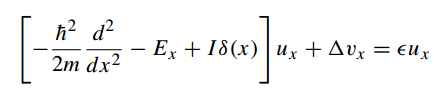• #### ​例2：一个 $ϵ >Δ$ 的电子从正常态入射

1. 类似无绝缘层Andeev共有四种情况。

• #### ​多重安德列夫反射MAR(次谐波能隙结构Subharmonic energy-gap structure )PRB.27,6739

1. 在无散射情况z=0下，当温度接近0，次谐波能隙结构逐渐消失。也就是说尽管多重安德列夫反射导致次谐波能隙结构，也需要在正常反射的作用下放大后才能在低温下观测到。

• #### ​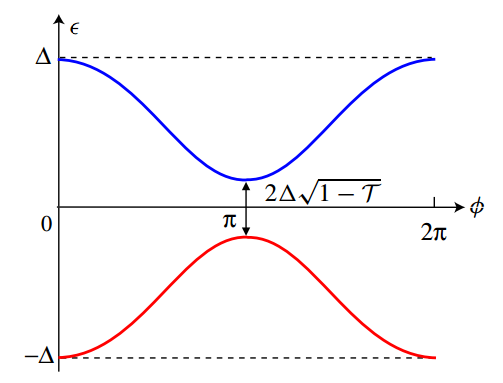1. 界面势垒使得镜面反射存在，导致向左运动和向右运动的两支能带耦合而打开gap。
2. 当T=1时，变成完全Andeev反射。
3. 当T~0时，gap贴合体能隙。

• #### ​ $\frac{1}{ R_{N} } = \frac{T}{ R_{sh} }$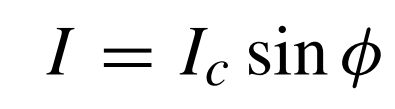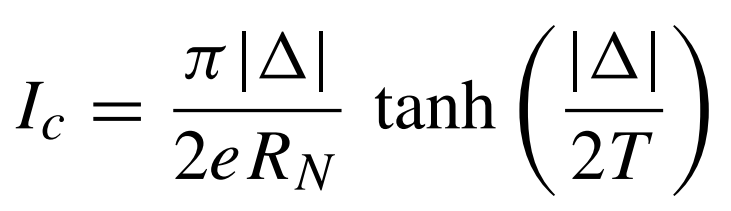1. 电导越小，超流相位关系越接近正弦函数。
2. Josephson 结可视为两个超导体之间的弱耦合。因此超流相位关系为正弦函数。

• #### ​DC Josephson effect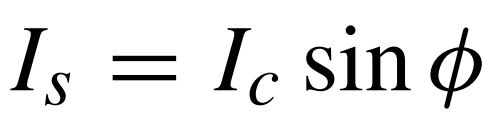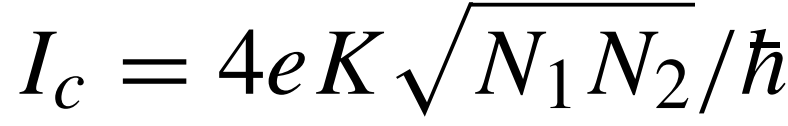K是耦合能，N1，N2是库珀对数量。

这里的Ic就是SIS结中透射率远小于1时候的Ic.

• #### ​Josephson 自由能： $F_{J} = E_{J} (1-cos( ϕ )) ​$ ; $E_{J} = \frac{ℏI_{c} }{2e}$

1. 自由能：相对于没有超流时候的能量。
2. 自由能与驻波的能量相位关系是不同的。自由能是实际的能量。能量相位关系相当于能谱。
• #### ​AC Josephson effect:  由功率关系 $\frac{dF_{J} }{dt} = I_{s} V$ 可得 :$ℏ ω _{J} =2eV; \newline ℏ \frac{d ϕ }{dt} =2eV$

1. 此功率关系没有考虑Josephson 结的正常电阻和电容。
• #### ​Josephson 电感L，依赖于结两端的相位。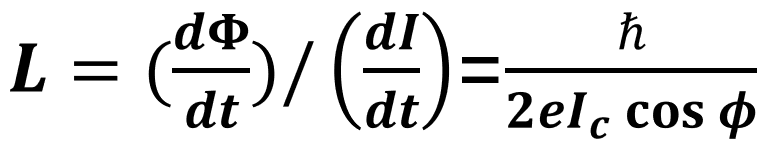感生电动势与电流变化率成正比，比例系数就是电感。